# Introduction To Determinants

Determinants are very important concept related to square matrix, and usually it is simple to calculate one while dealing with the system of linear equations. Our attempt here is to understand determinants properly so that all related concepts becomes easy and unforgettable. So we begin with a gentle introduction to determinants of matrices.

### What are Determinants ?

Imagine there are no matrices and you must solve the system of linear equation with simple algebra. For example,

Consider the following system of linear equation with just one equation.

a_{11}x_1 = b_1

To solve this equation using algebra method divide both sides by constant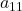.

x_1 = b_1/a_{11}

The elementis the determinant in the equation as a denominator. Provided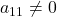. Otherwise, the system has no solution.

A determinant is zero does not always means that the system of linear equation has no solution, sometimes it has infinite solutions. But, if determinant is 0, then the matrix is not invertible.

Let us take another example, where there are two linear system of equations with two unknowns.

\begin{aligned}
&a_{11}x_1 + a_{12}x_2 = b_1\\\\
&a_{21}x_1 + a_{22}x_2 = b_2
\end{aligned}

When we solve for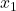the equations become

\begin{aligned}
&x_1 = (b_1 - a_{12}x_2)/a_{11}\\\\
&x_1 = (b_2 - a_{22}x_2)/a_{21}
\end{aligned}

Comparing both equations we get,

\begin{aligned}
&x_1 = a_{21}b_1 - a_{12}a_{21} = a_{11}b_2 - a_{11}a_{22}\\\\
&= a_{21}b_1 - a_{11}b_2 = a_{12}a_{21} - a_{11}a_{22}\\\\
&= (a_{21}b_1 - a_{11}b_2)/(a_{12}a_{21} - a_{11}a_{22})
\end{aligned}

The denominator should be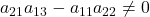, else the equation will collapse.

### Generalizing the Denominator

From the above result, there is a pattern to the denominator and if you solve for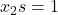then you will find out that the denominator is not changing and it remain the same. Hence, the denominator is determinant of the equation given that it is not equal to zero.

For a system of two equations and two unknowns , the determinant is the following.

a_{12}a_{21} - a_{11}a_{22}

Observe that in any term of determinant, the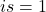corresponds to sequence of numbers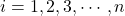and all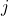in the term is a permutation of the sequence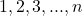. Therefore, we could generalize determinant as

= \sum \pm a_{1\alpha}a_{2\beta}...a_{nv}

where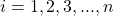determines the number of variables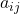in a term.

### Problem Of Negative And Positive Terms

Though we were able to generalize any term of the determinant with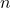variables in each term. Some terms are positive and some are negative. The next question is to determine which are positive and which are negative terms where any single terms is given by the following.

= \sum \pm a_{1\alpha}a_{2\beta}...a_{nv}

Before that you need to be familiar with following concepts.

#### Inversion and Transposition

In the determinant term,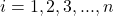in natural order and allare a permutation of the natural order (1,2,3,…,n).

Given a permutation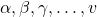if two indices are out of their natural order and greater index comes first and then lesser index, then it is called inversion.

Suppose that the natural order is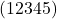and the permutation is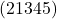, here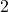andis inversion as they are out of the natural order.

Suppose that the natural order is disturbed by swapping two numbers. If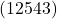is the permutation then only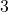and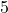is swapped. This is called transposition.

The number of inversion is unique and can be counted. If the inversions are even then permutation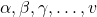belongs to even class, otherwise, odd class.

If a term belongs to odd class, then assign negative sign, else assign a positive sign if it belongs to even class.

### Determinant of 3 x 3 Equations

Using the information above let us systematically find determinant of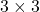equations. Since,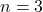there will be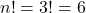terms with each term having 3 variables that is,.

Write down each permutation and the term of determinant.

\begin{aligned}
&a_{11}a_{22}a_{33}  \hspace{5px} //permutation \hspace{5px} of \hspace{5px} j \hspace{5px} is\hspace{5px} (1 2 3)\\\\
&a_{11}a_{23}a_{32} \hspace{5px} //permutation \hspace{5px}of \hspace{5px} j \hspace{5px} is \hspace{5px} (1 3 2)\\\\
&a_{12}a_{21}a_{33} \hspace{5px} //permutation \hspace{5px}of \hspace{5px} j \hspace{5px} is \hspace{5px}(2 1 3)\\\\
&a_{12}a_{23}a_{31} \hspace{5px} //permutation \hspace{5px} of \hspace{5px} j \hspace{5px}is \hspace{5px} (2 3 1)\\\\
&a_{13}a_{21}a_{32} \hspace{5px} //permutation \hspace{5px} of \hspace{5px} j \hspace{5px} is \hspace{5px} (3 1 2)\\\\
&a_{13}a_{22}a_{31} \hspace{5px} //permutation \hspace{5px} of \hspace{5px} j \hspace{5px} is \hspace{5px} (3 2 1)
\end{aligned}

We get following,

a_{11}a_{22}a_{33}+a_{11}a_{23}a_{32}+a_{12}a_{21}a_{33}+a_{12}a_{23}a_{31}a_{13}a_{21}a_{32}+a_{13}a_{22}a_{31}

We must look at the permutation and change the sign of terms that belong to odd class meaning permutations that have odd number of inversions.

= a_{11}a_{22}a_{33}-a_{11}a_{23}a_{32}-a_{12}a_{21}a_{33}+a_{12}a_{23}a_{31}a_{13}a_{21}a_{32}-a_{13}a_{22}a_{31}

This is a faster method of finding determinants, but you must be careful in checking the number of inversions. Next article, we shall discuss about finding determinants by cross multiplications and some interesting properties of determinants.